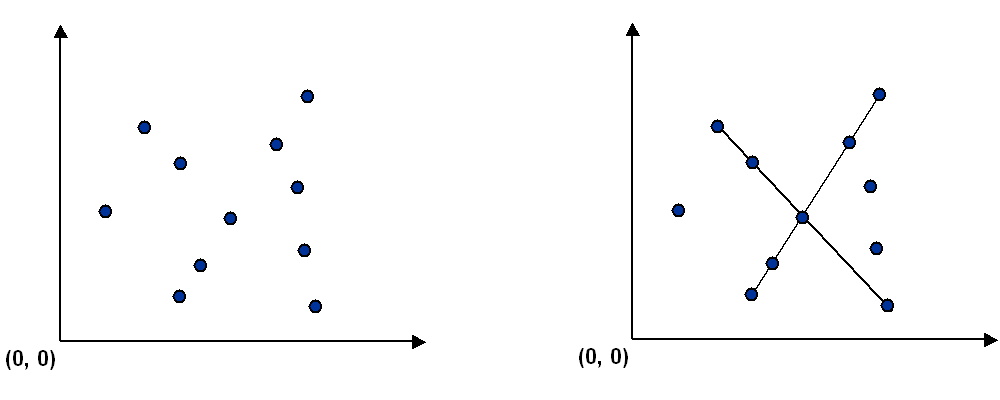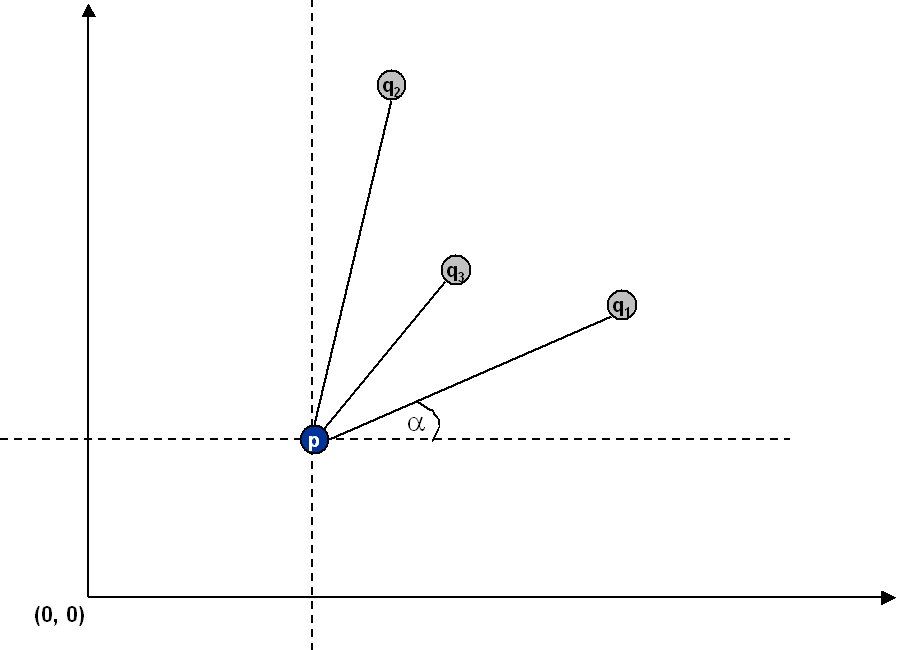## Pattern Recognition

Write a program to recognize line patterns in a given set of points.

Computer vision involves analyzing patterns in visual images and reconstructing the real world objects that produced them. The process in often broken up into two phases: feature detection and pattern recognition. Feature detection involves selecting important features of the image; pattern recognition involves discovering patterns in the features. We will investigate a particularly clean pattern recognition problem involving points and line segments. This kind of pattern recognition arises in many other applications, for example statistical data analysis.

The problem. Given a set of N feature points in the plane, determine every line segment that contains 4 or more of the points, and plot all such line segments.Brute force. Write a program brute.c that examines 4 points at a time and checks if they all lie on the same line segment, plotting any such line segments in turtle graphics. To get started, first implement a data type for points in the plane. The file point.c implements the Point interface defined in point.h. You will need to supply additional interface functions to support the brute force client, e.g., checking whether three points lie on the same line. You can begin your brute force client from the the client plotpoints.c, which reads in a list of points and plots them using turtle graphics.

A sorting solution. Remarkably, it is possible to solve the problem much faster than the brute force solution described above. Given a point p, the following method determines whether p participates in a group of 4 or more collinear points. Applying this method for each of the N points in turn yields an efficient algorithm to the problem.

• Think of p as the origin.

• For each point q, determine the angle it makes with p.

• Sort the points according to the angles they makes with p.

• Check if any 3 adjacent points in the sorted order have equal angles with p. If so, these points, together with p, are collinear.The algorithm works because points that make the same angle with p are collinear and sorting brings such points together. The algorithm is fast because the bottleneck operation is sorting.

Input format. Assume the points are given as pairs of integers (x, y) between 0 and 32,768.

```16384  19200
16384  21120
16384  32000
16384  21761
10000  10000
```

Output format. Your program should output a turtle graphics program that draws all of the points and the line segments you discover, as in the output below. Recall the command "F x y" sends the turtle to coordinate (x, y). The command "S d" draws a spot of size d, centered at the current location. The command "G x y" draws a line from the current location to (x, y), leaving the turtle at (x, y). Note that the points are scaled down by a factor of 64.0 so that they fit inside the 512-by-512 turtle graphics window.

```F 256.000000 300.000000 S 2
F 256.000000 330.000000 S 2
F 256.000000 500.000000 S 2
F 256.000000 340.015625 S 2
F 156.250000 156.250000 S 2

F 256.000000 330.000000 G 256.000000 500.000000
```
To view the result, compile turtle.c into an executable turtle, type "a.out < data.txt | turtle > data.eps" to create the PostScript file, and view with any PostScript viewer.

Analysis. Estimate the running time of your two programs as a function of N. Provide analytical and empirical evidence to support your answer.